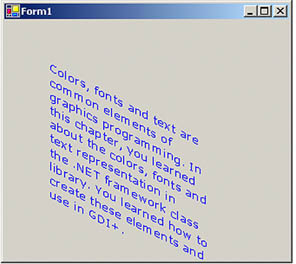# Text Transformation

In Chapter 5 we discussed how to use the ScaleTransform, RotateTransform, and TranslateTransform methods to transform text. We can also use a transformation matrix to transform text.

We create a Matrix object with the transformation properties and apply it to the surface using the Transform property of the Graphics object. Listing 10.21 creates a Matrix object and sets it as the Transform property. We then call DrawString, which draws the text on the form. To test this code, add the code to a form's paint event handler.

Listing 10.21 Text transformation example

```Graphics g = e.Graphics;
string str =
"Colors, fonts, and text are common" +
" elements of graphics programming." +
"In this chapter, you learned " +
" about the colors, fonts, and text" +
" representations in the "+
".NET Framework class library. "+
"You learned how to create "+
"these elements and use them in GDI+.";
// Create a Matrix object
Matrix M = new Matrix(1, 0, 0.5f, 1, 0, 0);
g.RotateTransform(45.0f,
System.Drawing.Drawing2D.MatrixOrder.Prepend);
g.TranslateTransform(-20, -70);
g.Transform = M;
g.DrawString(str,
new Font("Verdana", 10),
new SolidBrush(Color.Blue),
new Rectangle(50,20,200,300) );
```

Figure 10.30 shows the outcome of Listing 10.21.

Figure 10.30. Using the transformation matrix to transform textWe can apply shearing and other effects by changing the values of Matrix. For example, if we change Matrix as follows:

```
```
```Matrix M = new Matrix(1, 0.5f, 0, 1, 0, 0);
```
```
```

the new code will generate Figure 10.31.

Figure 10.31. Using the transformation matrix to shear textWe can reverse the text just by changing the value of the Matrix object as follows:

```
```
```Matrix M = new Matrix(1, 1, 1, -1, 0, 0);
```
```
```

with the results shown in Figure 10.32.

Figure 10.32. Using the transformation matrix to reverse textGDI+ Programming with C#
ISBN: 073561265X
EAN: N/A
Year: 2003
Pages: 145